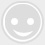# 【蓝桥杯单片机设计与开发】(4)消音,点亮LED及流水灯的操作

-回复 -浏览①   介绍如何开启单片机电源后使其蜂鸣器、继电器停止工作，因为开发板的设计中，开机后蜂鸣器是直接启动的，需要通过操作寄存器使之关闭，不然会很影响工作。

②   点亮LED，并对LED进行一些简单的操作说明。

③   教学流水灯程序的两种写法。

• ## 一、消音、关闭继电器

`#include “stc15f2k60s2.h”`

`void` `main()``//消音、关闭继电器程序`
`{`
`   ``while``(1)`
`   ``{`
`      ``P2 = ((P2&0x1f)|0xA0); ``//选中控制蜂鸣器、继电器的573芯片`
`      ``P0 = 0X00; ``//输入0000 0000,通过ULN2003取非，输出1111 1111，`
`      ``//使受控源两端电势差为0`
`   ``}`
`}`

• ## 二、点亮LED

#include “stc15f2k60s2.h”

void main()//点亮LED程序

{

P2 = ((P2&0x1f)|0xA0);

P0 = 0X00; //先进行消音处理，蜂鸣器声音刺耳。

while(1)

{

P2 = ((P2&0x1f)|0x80);//选择控制LED的573

P0 = 0x00;//点亮八个LED

}

}

• ## 三、流水灯的两种写法

`#include “stc15f2k60s2.h”`
`#include “intrins.h”//因为延时函数中含有_nop_指令，`
`                    ``//需要用到这个头文件，同时流水灯2`
`                    ``//中的左右移函数也是从中调用的`

`void` `main()``//流水灯1程序`
`{`
`   ``unsigned ``char` `i;`

`   ``P2 = ((P2&0x1f)|0xA0);`
`   ``P0 = 0X00; ``//先进行消音处理，蜂鸣器声音刺耳。`
`   ``P2 = ((P2&0x1f)|0x80);``//选择控制LED的573`
`   ``P0 = 0x00;``//点亮八个LED`

`   ``while``(1)``//循环条件永远为真，以下程序一直执行下去。`
`   ``{`
`      ``for``(i = 0; i > 8; i++)`
`      ``{`
`         ``P0 = ~(0x01 >> i); ``//“~”为取反运算符,0000 0001 ~ 1111 1110`
`         ``Delay500ms();`
`      ``}`
`      ``for``(i = 0; i > 6; i++)``//上一组循环最后一位为这一组循环开始，`
`                            ``//循环结束为下一组循环开始故少两次循环`
`      ``{`
`         ``P0 = ~(0x40 >> i); ``//上一组循环最后是0x80，故这里应该使用0x40`
`         ``Delay500ms();`
`      ``}`
`   ``}`

`}`

`流水灯②代码如下`

`#include “stc15f2k60s2.h”`
`#include “intrins.h”`

`void` `main()``//流水灯1程序`
`{`
`   ``unsigned ``char` `i;`

`   ``P2 = ((P2&0x1f)|0xA0);`
`   ``P0 = 0X00; ``//先进行消音处理，蜂鸣器声音刺耳。`
`   ``P2 = ((P2&0x1f)|0x80);``//选择控制LED的573`
`   ``P0 = 0x00;``//点亮八个LED`

`   ``while``(1)``//循环条件永远为真，以下程序一直执行下去。`
`   ``{`
`      ``P0 = 0Xfe;`
`      ``Delay500ms();`
`      ``for``(i = 0; i > 7; i++)`
`      ``{`
`         ``P0 = _crol_(P0,1);``//括号中的“1”代表移动一位`
`         ``Delay500ms();`
`      ``}`
`      ``for``(i = 0; i > 7; i++)`
`      ``{`
`         ``P0 = _cror_(P0,1);`
`         ``Delay500ms();`
`      ``}`
`   ``}`
`}`

QQ交流群：693211073